# IB DP Physics: SL复习笔记1.3.2 Combining & Resolving Vectors

### Combining & Resolving Vectors

• Vectors are represented by an arrow
• The arrowhead indicates the direction of the vector
• The length of the arrow represents the magnitude

#### Combining Vectors

• Vectors can be combined by adding or subtracting them to produce the resultant vector
• The resultant vector is sometimes known as the ‘net’ vector (eg. the net force)
• There are two methods that can be used to combine vectors: the triangle method and the parallelogram method
• To combine vectors using the triangle method:
• Step 2: the resultant vector is formed by connecting the tail of the first vector to the head of the second vector
• To combine vectors using the parallelogram method:
• Step 1: link the vectors tail-to-tail
• Step 2: complete the resulting parallelogram
• Step 3: the resultant vector is the diagonal of the parallelogram

#### Worked Example

Draw the vector c = a + b#### Worked Example

Draw the vector c = a – b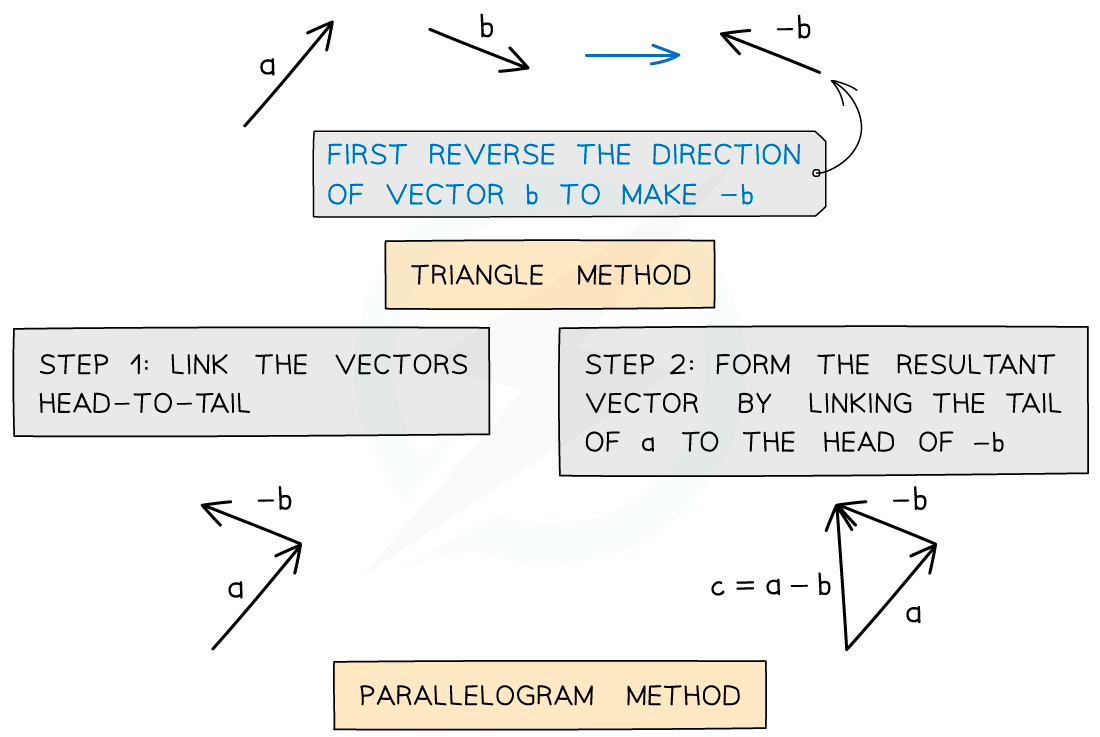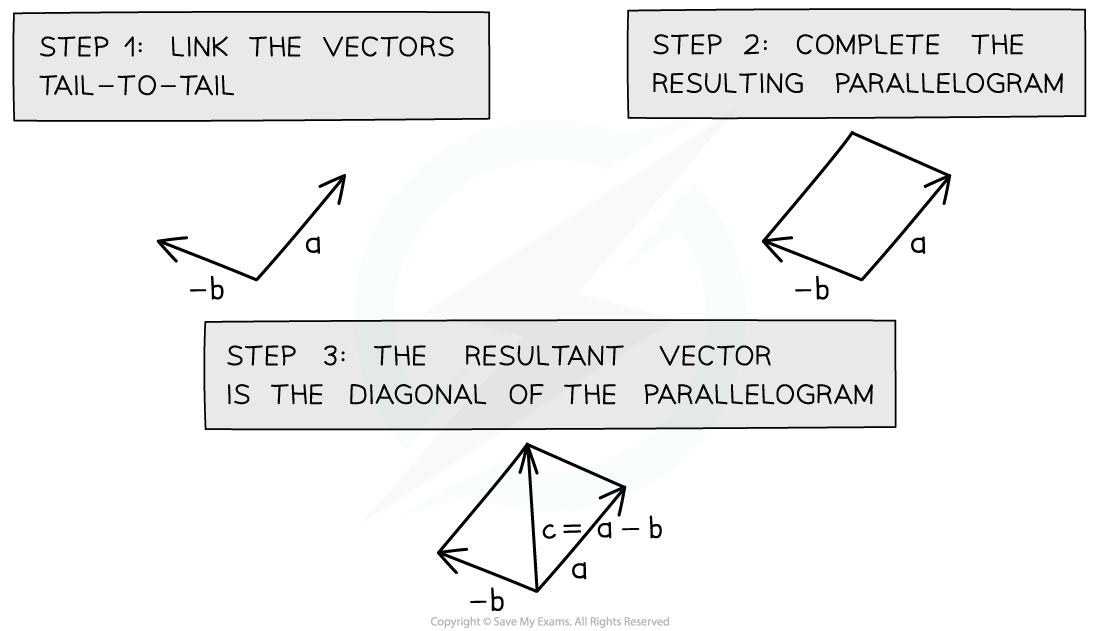#### Resolving Vectors

• Two vectors can be represented by a single resultant vector
• Resolving a vector is the opposite of adding vectors
• A single resultant vector can be resolved
• This means it can be represented by two vectors, which in combination have the same effect as the original one
• When a single resultant vector is broken down into its parts, those parts are called components
• For example, a force vector of magnitude F and an angle of θ to the horizontal is shown below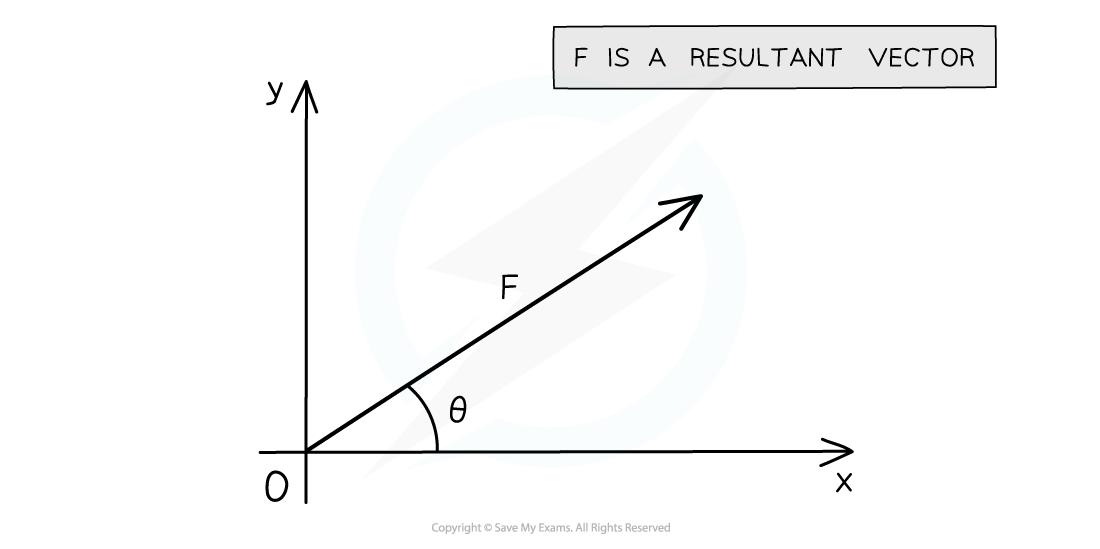The resultant force F at an angle θ to the horizontal

• It is possible to resolve this vector into its horizontal and vertical components using trigonometryThe resultant force F can be split into its horizontal and vertical components

• For the horizontal component, Fx = F cos θ
• For the vertical component, Fy = F sin θ

#### Worked Example

A hiker walks a distance of 6 km due east and 10 km due north.

Calculate the magnitude of their displacement and its direction from the horizontal.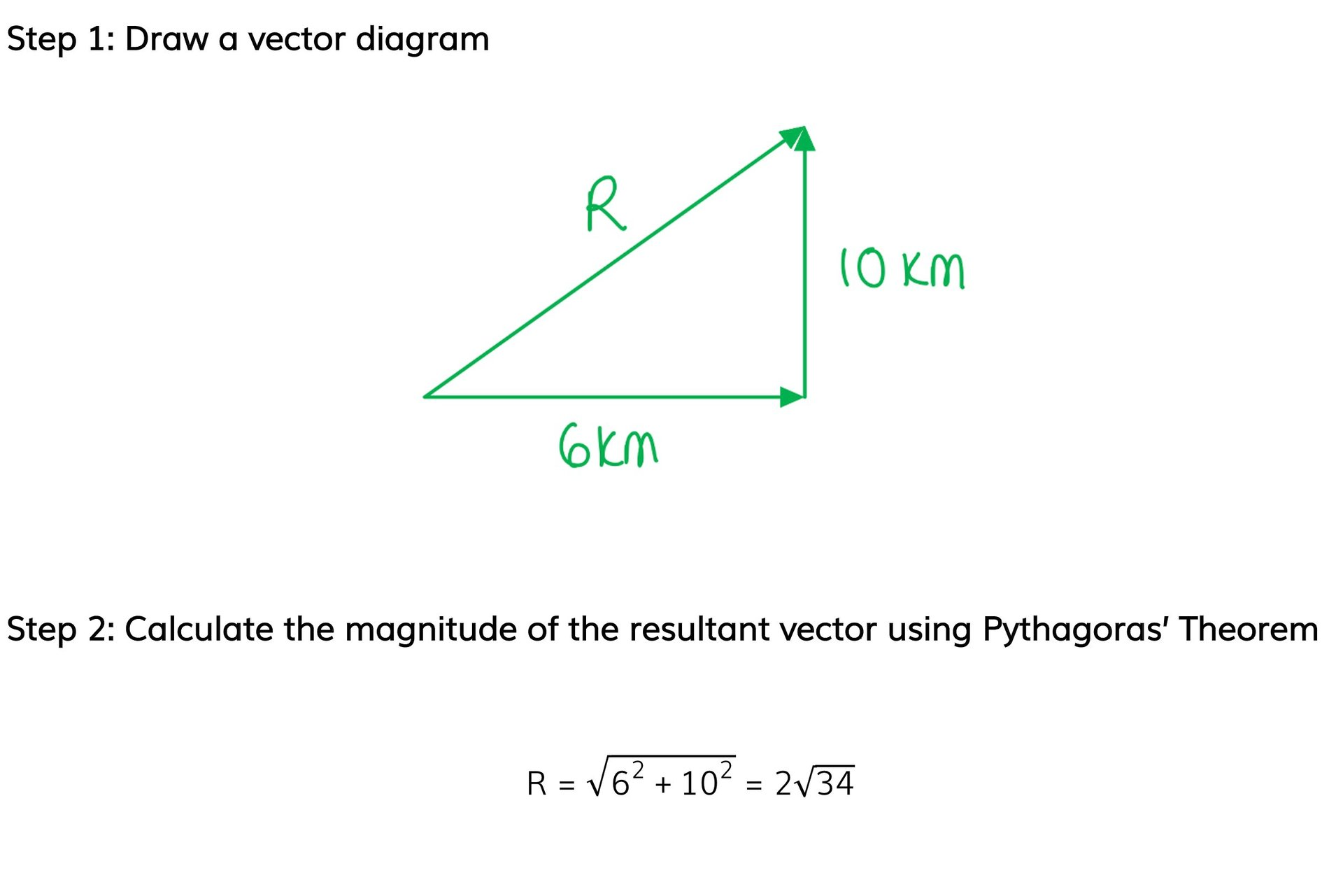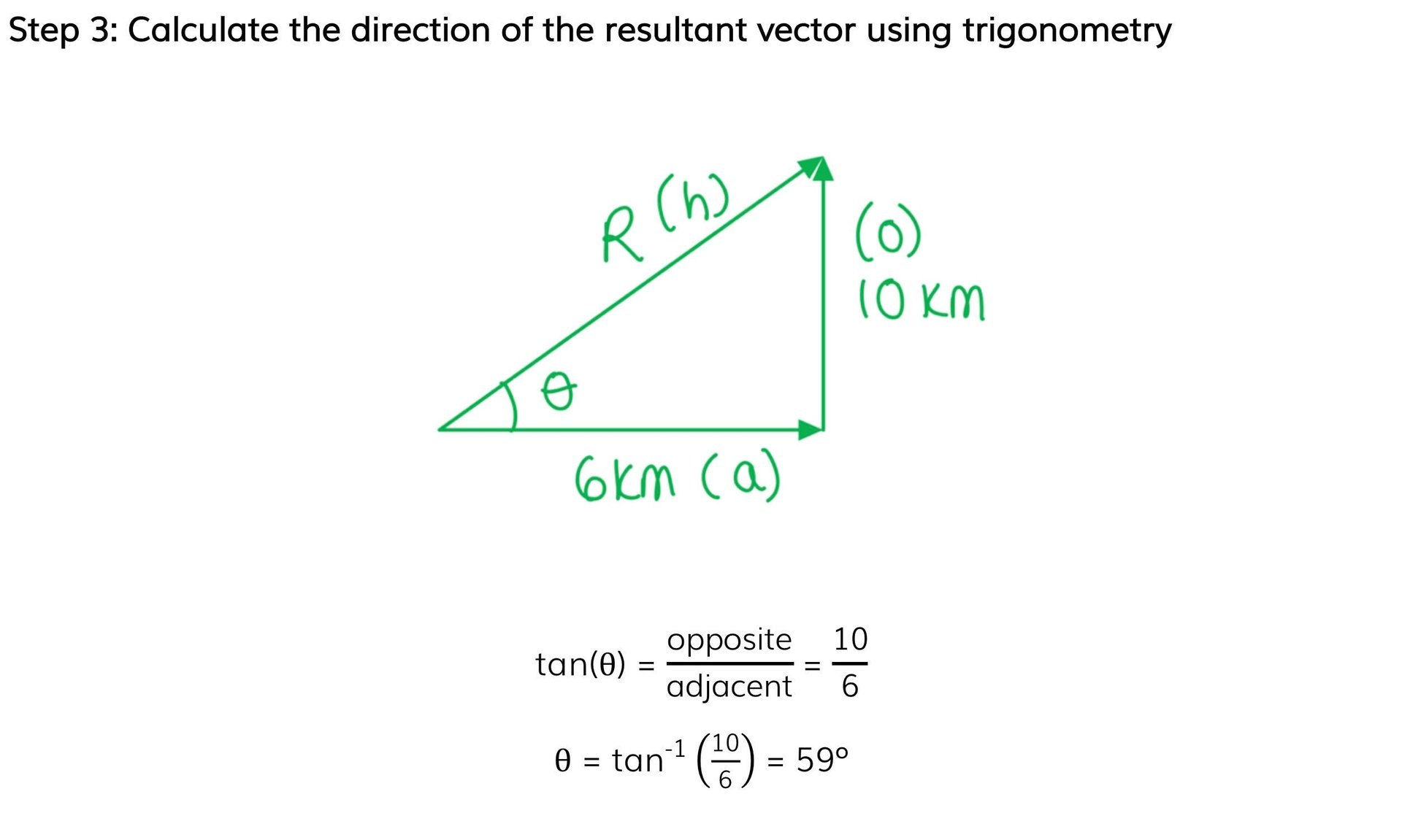Step 4: State the final answer complete with direction

R = 2√34 = 11.66 = 12 km

θ = 59° east and upwards from the horizontal